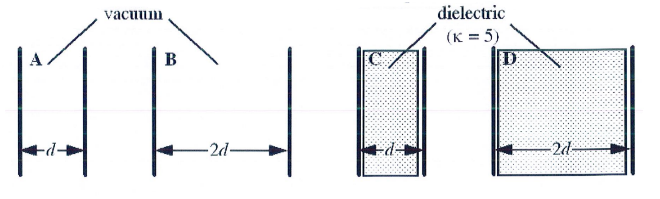# Problem: The figure below shows four parallel plate capacitors: A, B, C, and D. Each capacitor carries the same charge q and has the same plate area A. As suggested by the figure, the plates of capacitor A and C are separated by a distance  d while those of B and D are separated by a distance 2d. Capacitors A and B are maintained in vacuum while capacitors C and D contain dieelctrics with constant k = 5. Which list below places the capacitors in order of  increasing capacitance?  A) A, B, C, D B) B, A, C, D C) A, B, D, C D) B, A, D, C E) D, C, B, A

###### FREE Expert Solution
96% (115 ratings)
###### Problem Details

The figure below shows four parallel plate capacitors: ABC, and D. Each capacitor carries the same charge q and has the same plate area A. As suggested by the figure, the plates of capacitor A and C are separated by a distance  d while those of B and D are separated by a distance 2d. Capacitors A and B are maintained in vacuum while capacitors C and D contain dieelctrics with constant k = 5. Which list below places the capacitors in order of  increasing capacitance?

A) A, B, C, D

B) B, A, C, D

C) A, B, D, C

D) B, A, D, C

E) D, C, B, AFrequently Asked Questions

What scientific concept do you need to know in order to solve this problem?

Our tutors have indicated that to solve this problem you will need to apply the Intro To Dielectrics concept. You can view video lessons to learn Intro To Dielectrics. Or if you need more Intro To Dielectrics practice, you can also practice Intro To Dielectrics practice problems.

How long does this problem take to solve?

Our expert Physics tutor, Juan took 3 minutes and 35 seconds to solve this problem. You can follow their steps in the video explanation above.

What professor is this problem relevant for?

Based on our data, we think this problem is relevant for Professor Jerousek's class at UCF.